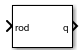# Rodrigues to Quaternions

Convert Euler-Rodrigues vector to quaternion

• Library:
• Aerospace Blockset / Utilities / Axes Transformations

•## Description

The Rodrigues to Quaternions block determines the 4-by-1 quaternion from a three-element Euler-Rodrigues vector. Aerospace Blockset™ uses quaternions that are defined using the scalar-first convention. For more information on Euler-Rodrigues vectors, see Algorithms.

## Ports

### Input

expand all

Euler-Rodrigues vector from which to determine the quaternion.

Data Types: `double`

### Output

expand all

Quaternion determined from the Euler-Rodrigues vector.

Data Types: `double`

## Algorithms

An Euler-Rodrigues vector $\stackrel{⇀}{b}$ represents a rotation by integrating a direction cosine of a rotation axis with the tangent of half the rotation angle as follows:

`$\stackrel{\to }{b}=\left[\begin{array}{ccc}{b}_{x}& {b}_{y}& {b}_{z}\end{array}\right]$`

where:

`$\begin{array}{l}{b}_{x}=\mathrm{tan}\left(\frac{1}{2}\theta \right){s}_{x},\\ {b}_{y}=\mathrm{tan}\left(\frac{1}{2}\theta \right){s}_{y},\\ {b}_{z}=\mathrm{tan}\left(\frac{1}{2}\theta \right){s}_{z}\end{array}$`

are the Rodrigues parameters. Vector $\stackrel{⇀}{s}$ represents a unit vector around which the rotation is performed. Due to the tangent, the rotation vector is indeterminate when the rotation angle equals ±pi radians or ±180 deg. Values can be negative or positive.

 Dai, J.S. "Euler-Rodrigues formula variations, quaternion conjugation and intrinsic connections." Mechanism and Machine Theory, 92, 144-152. Elsevier, 2015.